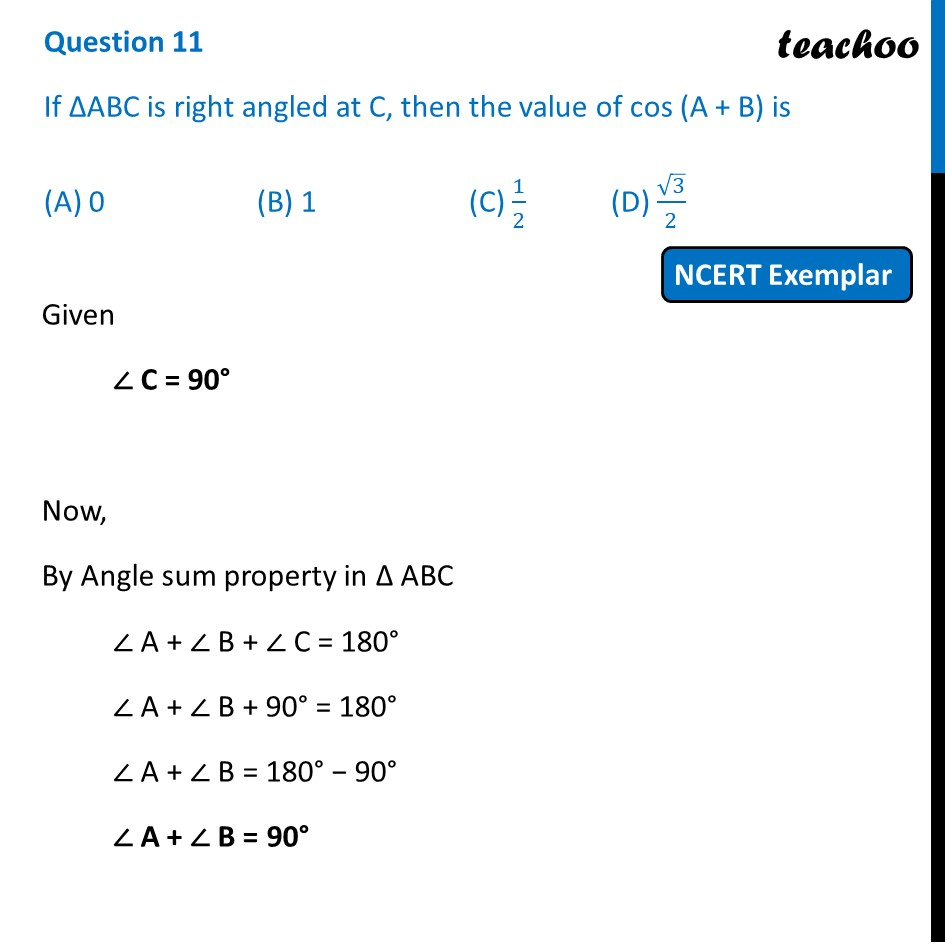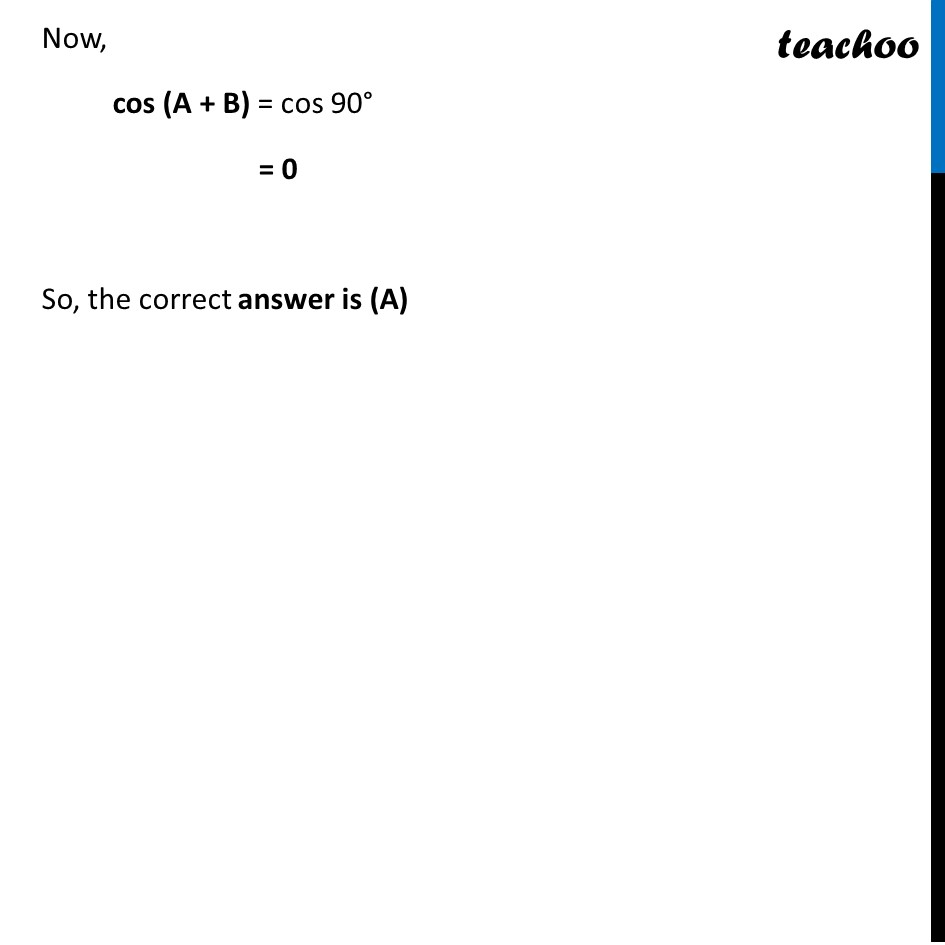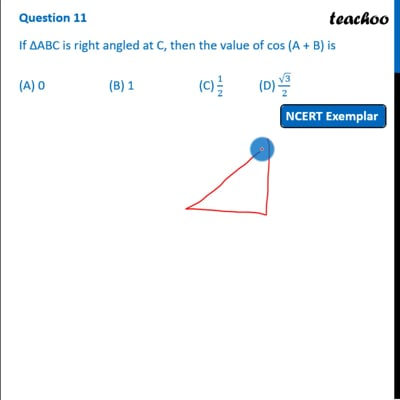NCERT Exemplar - MCQ

Chapter 8 Class 10 Introduction to Trignometry
Serial order wise

## (A) 0   (B) 1   (C) 1/2    (D) √3/2This video is only available for Teachoo black users

Maths Crash Course - Live lectures + all videos + Real time Doubt solving!

### Transcript

Question 11 If ∆ABC is right angled at C, then the value of cos (A + B) is (A) 0 (B) 1 (C) 1/2 (D) √3/2 Given ∠ C = 90° Now, By Angle sum property in Δ ABC ∠ A + ∠ B + ∠ C = 180° ∠ A + ∠ B + 90° = 180° ∠ A + ∠ B = 180° − 90° ∠ A + ∠ B = 90° Now, cos (A + B) = cos 90° = 0 So, the correct answer is (A)